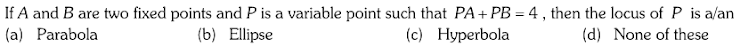CHECK POINTS ON BASICS COORDINATE GEOMETRY
There is no negative marking. 4 marks will be given for every correct answer. Good luck.
NAME *
MOBILE NUMBER *
Q.1 Cartesian Co-ordinates of a Point, Polar Co-ordinates, Distance Formula
4 points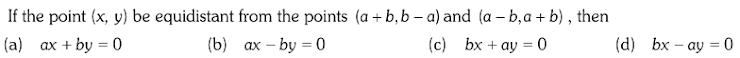Q.2 Cartesian Co-ordinates of a Point, Polar Co-ordinates, Distance Formula
4 points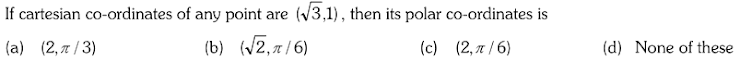Q.3 Geometrical Conditions.(1) Properties of triangles (2) Properties of quadrilaterals
4 points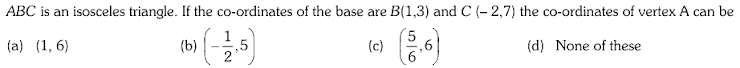Q.4 Geometrical Conditions.(1) Properties of triangles (2) Properties of quadrilaterals
4 points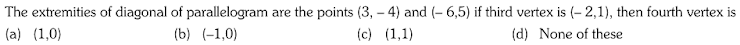Q.5 Geometrical Conditions.(1) Properties of triangles (2) Properties of quadrilaterals
4 points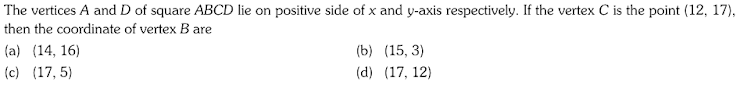Q.6 Geometrical Conditions.(1) Properties of triangles (2) Properties of quadrilaterals
4 points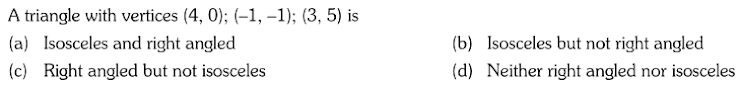Q.7 Section Formulae.(1) Internal division : (2) External division :
4 points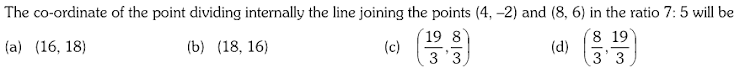Q.8 Section Formulae.(1) Internal division : (2) External division :
4 points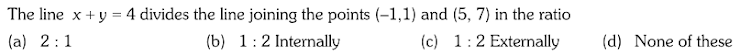Q.9 Section Formulae.(1) Internal division : (2) External division :
4 points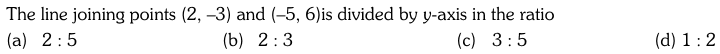Q.10 Some points of a Triangle.(1) Centroid of a triangle :
4 points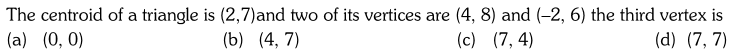Q.11 (2) Circumcentre :
4 points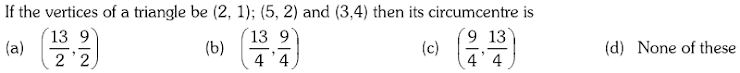Q.12 (3) Incentre : (4) Excircle :
4 points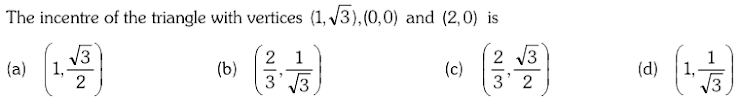Q.13 (5) Orthocentre :
4 points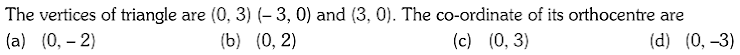Q.14 (5) Orthocentre :
4 points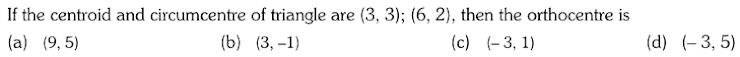Q.15 Area of some Geometrical figures.(1) Area of a triangle : (2) Collinear points : (3) Area of a quadrilateral : (4) Area of polygon :
4 points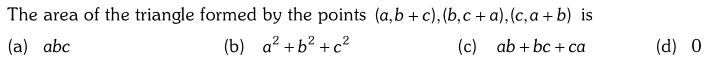Q.16 Area of some Geometrical figures.(1) Area of a triangle : (2) Collinear points : (3) Area of a quadrilateral : (4) Area of polygon :
4 points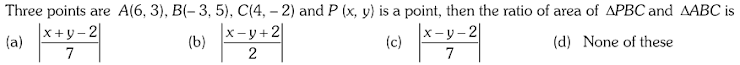Q.17 Area of some Geometrical figures.(1) Area of a triangle : (2) Collinear points : (3) Area of a quadrilateral : (4) Area of polygon :
4 points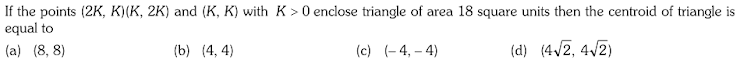Q.18 Area of some Geometrical figures.(1) Area of a triangle : (2) Collinear points : (3) Area of a quadrilateral : (4) Area of polygon :
4 points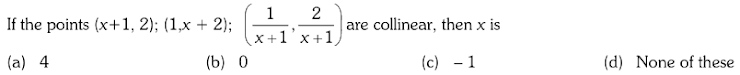Q.19 Area of some Geometrical figures.(1) Area of a triangle : (2) Collinear points : (3) Area of a quadrilateral : (4) Area of polygon :
4 points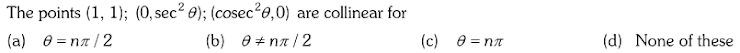Q.20 Area of some Geometrical figures.(1) Area of a triangle : (2) Collinear points : (3) Area of a quadrilateral : (4) Area of polygon :
4 points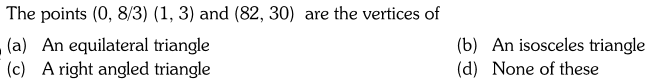Q.21 Transformation of Axes .(1) Shifting of origin without rotation of axes (2) Rotation of axes without changing the origin : (3) Change of origin and rotation of axes (4) Reflection (Image of a point)
4 points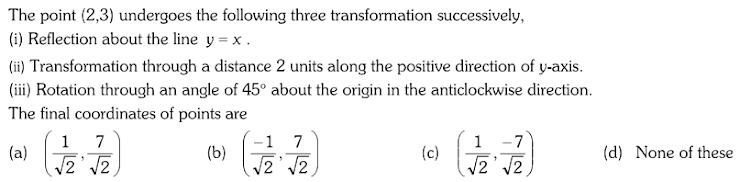Q.22 Transformation of Axes .(1) Shifting of origin without rotation of axes (2) Rotation of axes without changing the origin : (3) Change of origin and rotation of axes (4) Reflection (Image of a point)
4 points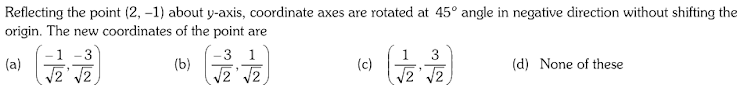Q.23 Transformation of Axes .(1) Shifting of origin without rotation of axes (2) Rotation of axes without changing the origin : (3) Change of origin and rotation of axes (4) Reflection (Image of a point)
4 points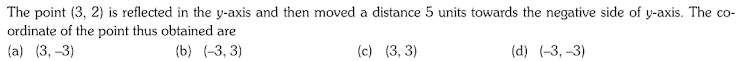Q.24 Locus.
4 points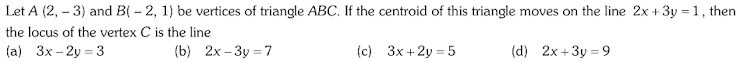Q.25 Locus.
4 points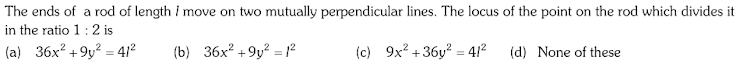Q.26 Locus.
4 points